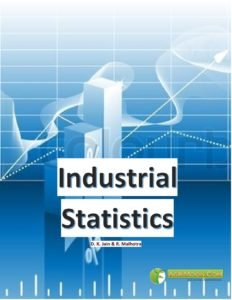You are here
Home > Book > Industrial Statistics ICAR ecourse PDF Book Free Download

Book Detail: Industrial StatisticsLanguage: English

Pages: 236

Author: D. K. Jain & R. Malhotra

Price: FreeCourse outline: Industrial Statistics

Module 1: Descriptive Statistics
Lesson 1. Definition and Scope of Statistics
Lesson 2. Classification of Data and Frequency Distribution
Lesson 3. Measures of Central Tendency
Lesson 4. Measures of Dispersion
Lesson 5. Measures of Skewness and Kurtosis

Module 2: Theory of Probability
Lesson 6. Elementary Notions of Probability
Lesson 7. Addition Theorem of Probability
Lesson 8. Multiplication Theorem of Probability

Module 3: Probability Distributions
Lesson 9. Random Variable and its Probability Distribution
Lesson 10. Binomial Distribution
Lesson 11. Poisson Distribution
Lesson 12. Normal Distribution

Module 4: Concepts of Sampling Methods
Lesson 13. Sampling Theory and Sampling Distribution
Lesson 14. Simple Random Sampling
Lesson 15. Elementary Concepts of other Sampling Techniques

Module 5: Test of Significance
Lesson 16. Testing of Hypothesis
Lesson 17. Z – test and its applications
Lesson 18. t – test and its applications
Lesson 19. Chi – Square test and its applications
Lesson 20. F – test and its applications

Module 6: Analysis of Variance
Lesson 21. One Way Classification
Lesson 22. Two Way Classification

Module 7: Correlation and Regression
Lesson 23. Linear Correlation
Lesson 24. Rank Correlation
Lesson 25. Linear Regression

Module 8: Statistical Quality Control
Lesson 26. Basic Concept of Statistical Quality Control
Lesson 27. Control Charts for Variables
Lesson 28. Control Charts for Attributes
Lesson 29. Fundamental Concepts of Acceptance Sampling Plan by Attributes
Lesson 30. OC and AOQ Curves

Disclaimer: The information on this website does not warrant or assume any legal liability or responsibility for the accuracy, completeness or usefulness of the courseware contents.

The contents are provided free for noncommercial purpose such as teaching, training, research, extension and self learning.